### Total 40 Videos found in Category "AP Statistics Skills"

Title
 1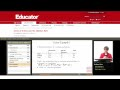Statistics Skills: Addition Rule for Mutually Exclusive Events 2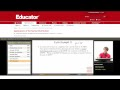Statistics Skills: Applications of the Normal Distribution 3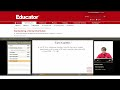Statistics Skills: Area Under a Normal Distribution Curve 4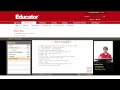Statistics Skills: Bayes Rule/Theorem 5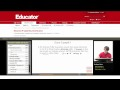Statistics Skills: Binomial Probability Distribution 6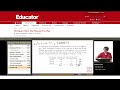Statistics Skills: Chi Squared Test 7Statistics Skills: Combining Independent Random Variables 8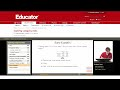Statistics Skills: Conditional Probabilities (Categorical Data) 9Statistics Skills: Confidence Intervals (Difference in Means) 10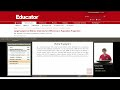Statistics Skills: Confidence Intervals (Difference in Population Proportion) 11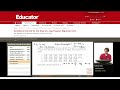Statistics Skills: Confidence Intervals (Slope of Least Squares Regression Line) 12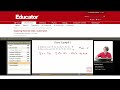Statistics Skills: Correlation (Bivariate Data) 13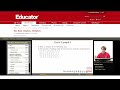Statistics Skills: Dotplots 14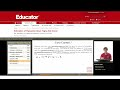Statistics Skills: Estimation of Population Mean (Sigma Not Known) 15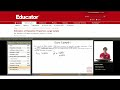Statistics Skills: Estimation of Population Proportion (Large Sample) 16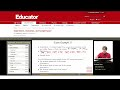Statistics Skills: Experiment, Outcomes, Sample Space 17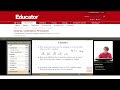Statistics Skills: Factorials, Combinations, Permutations 18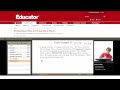Statistics Skills: Finding Values with Probability Known (Applications of the Normal Distribution) 19Statistics Skills: Geometric Probability Distribution 20Statistics Skills: Hypothesis Test for a Difference Between Two Means 21Statistics Skills: Hypothesis Test for a Mean 22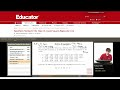Statistics Skills: Hypothesis Testing for Slope of a Least Squares Regression Line 23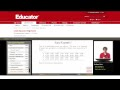Statistics Skills: Least Squares Regression 24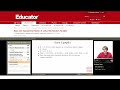Statistics Skills: Mean and Standard Deviation of a Discrete Random Variable 25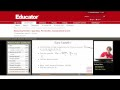Statistics Skills: Measuring Position (Quartile, Percentiles, Standardized Scores) 26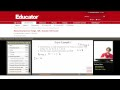Statistics Skills: Measuring Spread (Interquartile Range and Standard Deviation) 27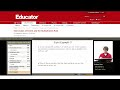Statistics Skills: Multiplication Rule for Independent Events 28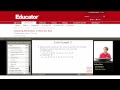Statistics Skills: Parallel Boxplots (Univariate Data) 29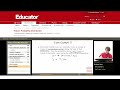Statistics Skills: Poisson Probability Distribution 30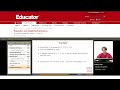Statistics Skills: Population and Sampling Distributions 31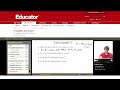Statistics Skills: Probability and Events 32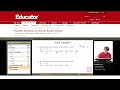Statistics Skills: Probability Distribution of a Discrete Random Variable 33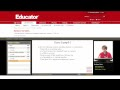Statistics Skills: Random Variables (Discrete or Continuous) 34Statistics Skills: Sample Size Estimation of a Population Mean 35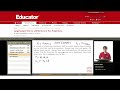Statistics Skills: Sample Test for a Difference in Two Proportions 36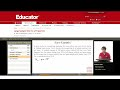Statistics Skills: Sample Test for a Proportion 37Statistics Skills: Sampling Distribution of a Sample Proportion (Central Limit Theorem) 38Statistics Skills: Sampling Distribution of the Sample Mean 39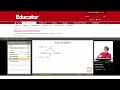Statistics Skills: Standard Normal Distribution (Z Scores) 40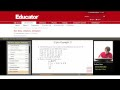Statistics Skills: Stemplot

Say and share some thing about these videos...